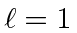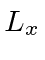## The Angular Momentum Matrices*

An important case of the use of the matrix form of operators is that of Angular Momentum Assume we have an atomic state with(fixed) butfree. We may use the eigenstates ofas a basis for our states and operators. Ignoring the (fixed) radial part of the wavefunction, our state vectors formust be a linear combination of thewhere, for example, is just the numerical coefficient of the eigenstate.

We will write our 3 component vectors likeThe angular momentum operators are therefore 3X3 matrices. We can easily derive the matrices representing the angular momentum operators for.(1)

The matrices must satisfy the same commutation relations as the differential operators.We verify this with an explicit computation of the commutator.

Since these matrices represent physical variables, we expect them to be Hermitian. That is, they are equal to their conjugate transpose. Note that they are also traceless.

As an example of the use of these matrices, let's compute an expectation value ofin the matrix representation for the general state.Jim Branson 2013-04-22